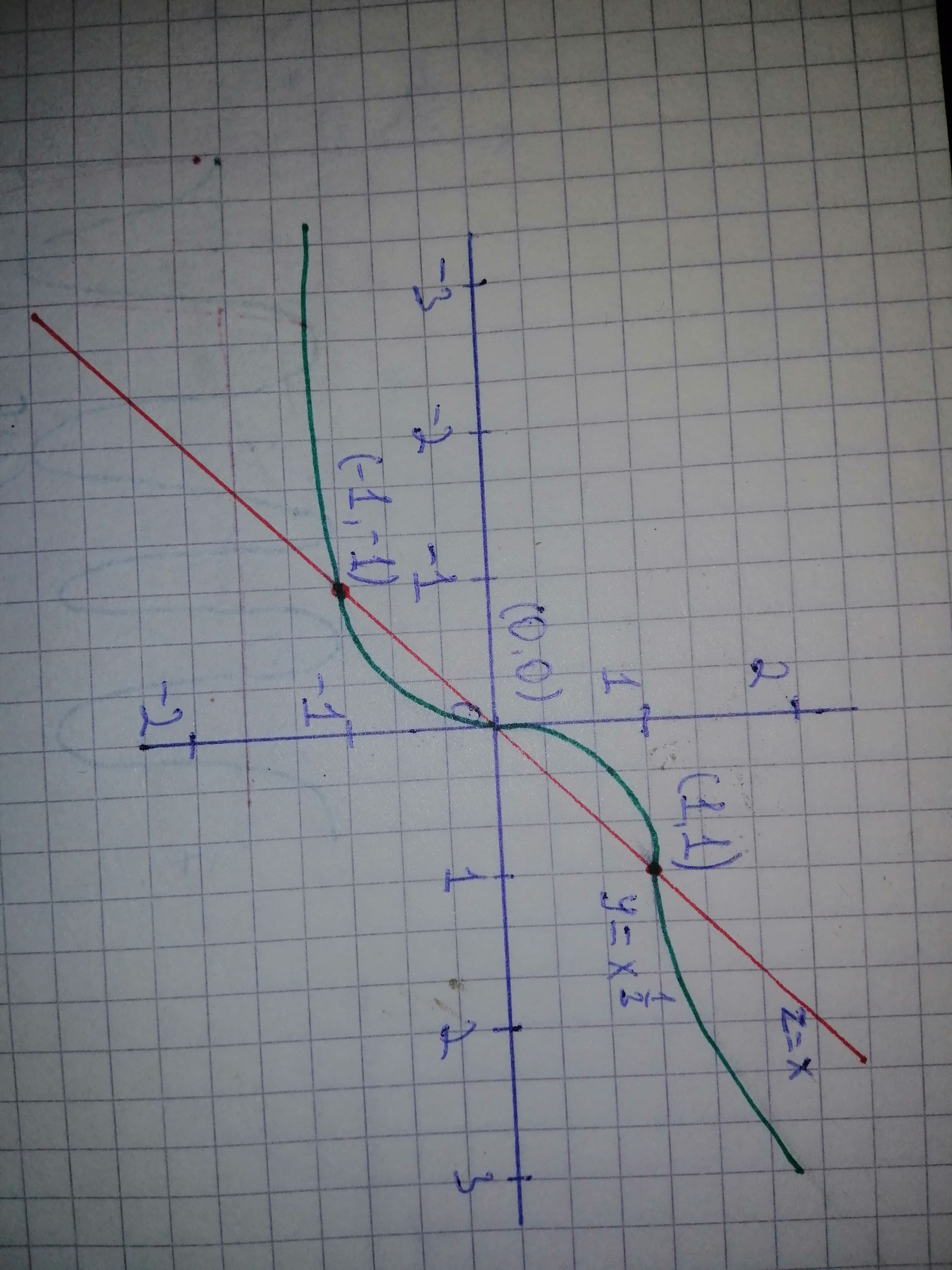# Solve the equation graphically in the given interval. State each answer rounded to two decimals. displaystyle{x}^{{frac{1}{{3}}}}-{x}={0},{left[-{3},{3}right]} x = ?generals336 2020-10-27 Answered
Solve the equation graphically in the given interval. State each answer rounded to two decimals.
${x}^{\frac{1}{3}}-x=0,\left[-3,3\right]$
$x=?$
You can still ask an expert for help

• Questions are typically answered in as fast as 30 minutes

Solve your problem for the price of one coffee

• Math expert for every subject
• Pay only if we can solve itDora

To find: Solve for x in the interval $\left[-3,3\right]$ graphically,
${x}^{\frac{1}{3}}-x=0$
$⇒{x}^{\frac{1}{3}}=x$
In order to solve graphically, we plot say and find the points where the two graphs intersect.From the graph it is clear that the graphs intersect at three points. That is, which are the solution for the given equation.
Hence, solution set is $\left\{-1,0,1\right\}.$